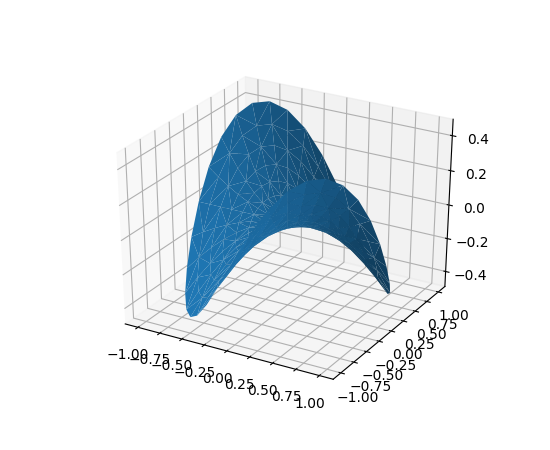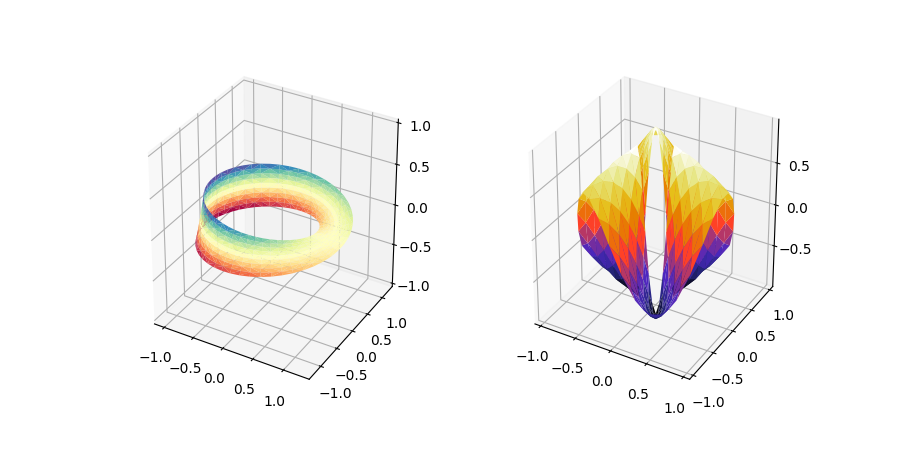Travis-CI:# The mplot3d Toolkit¶

Generating 3D plots using the mplot3d toolkit.

## Getting started¶

An Axes3D object is created just like any other axes using the projection=‘3d’ keyword. Create a new `matplotlib.figure.Figure` and add a new axes to it of type `Axes3D`:

```import matplotlib.pyplot as plt
from mpl_toolkits.mplot3d import Axes3D
fig = plt.figure()
ax = fig.add_subplot(111, projection='3d')
```

New in version 1.0.0: This approach is the preferred method of creating a 3D axes.

Note

Prior to version 1.0.0, the method of creating a 3D axes was different. For those using older versions of matplotlib, change `ax = fig.add_subplot(111, projection='3d')` to `ax = Axes3D(fig)`.

See the mplot3d FAQ for more information about the mplot3d toolkit.

### Line plots¶

`Axes3D.``plot`(xs, ys, *args, **kwargs)

Plot 2D or 3D data.

Argument Description
xs, ys x, y coordinates of vertices
zs z value(s), either one for all points or one for each point.
zdir Which direction to use as z (‘x’, ‘y’ or ‘z’) when plotting a 2D set.

Other arguments are passed on to `plot()`

### Scatter plots¶

`Axes3D.``scatter`(xs, ys, zs=0, zdir='z', s=20, c=None, depthshade=True, *args, **kwargs)

Create a scatter plot.

Argument Description
xs, ys Positions of data points.
zs Either an array of the same length as xs and ys or a single value to place all points in the same plane. Default is 0.
zdir Which direction to use as z (‘x’, ‘y’ or ‘z’) when plotting a 2D set.
s Size in points^2. It is a scalar or an array of the same length as x and y.
c A color. c can be a single color format string, or a sequence of color specifications of length N, or a sequence of N numbers to be mapped to colors using the cmap and norm specified via kwargs (see below). Note that c should not be a single numeric RGB or RGBA sequence because that is indistinguishable from an array of values to be colormapped. c can be a 2-D array in which the rows are RGB or RGBA, however, including the case of a single row to specify the same color for all points.
depthshade Whether or not to shade the scatter markers to give the appearance of depth. Default is True.

Keyword arguments are passed on to `scatter()`.

Returns a `Patch3DCollection`

### Wireframe plots¶

`Axes3D.``plot_wireframe`(X, Y, Z, *args, **kwargs)

Plot a 3D wireframe.

The `rstride` and `cstride` kwargs set the stride used to sample the input data to generate the graph. If either is 0 the input data in not sampled along this direction producing a 3D line plot rather than a wireframe plot. The stride arguments are only used by default if in the ‘classic’ mode. They are now superseded by `rcount` and `ccount`. Will raise ValueError if both stride and count are used.

` The `rcount` and `ccount` kwargs supersedes `rstride` and

`cstride` for default sampling method for wireframe plotting. These arguments will determine at most how many evenly spaced samples will be taken from the input data to generate the graph. This is the default sampling method unless using the ‘classic’ style. Will raise ValueError if both stride and count are specified. If either is zero, then the input data is not sampled along this direction, producing a 3D line plot rather than a wireframe plot. Added in v2.0.0.

Argument Description
X, Y, Data values as 2D arrays
Z
rstride Array row stride (step size), defaults to 1
cstride Array column stride (step size), defaults to 1
rcount Use at most this many rows, defaults to 50
ccount Use at most this many columns, defaults to 50

Keyword arguments are passed on to `LineCollection`.

Returns a `Line3DCollection`

### Surface plots¶

`Axes3D.``plot_surface`(X, Y, Z, *args, **kwargs)

Create a surface plot.

By default it will be colored in shades of a solid color, but it also supports color mapping by supplying the cmap argument.

The `rstride` and `cstride` kwargs set the stride used to sample the input data to generate the graph. If 1k by 1k arrays are passed in, the default values for the strides will result in a 100x100 grid being plotted. Defaults to 10. Raises a ValueError if both stride and count kwargs (see next section) are provided.

The `rcount` and `ccount` kwargs supersedes `rstride` and `cstride` for default sampling method for surface plotting. These arguments will determine at most how many evenly spaced samples will be taken from the input data to generate the graph. This is the default sampling method unless using the ‘classic’ style. Will raise ValueError if both stride and count are specified. Added in v2.0.0.

Argument Description
X, Y, Z Data values as 2D arrays
rstride Array row stride (step size)
cstride Array column stride (step size)
rcount Use at most this many rows, defaults to 50
ccount Use at most this many columns, defaults to 50
color Color of the surface patches
cmap A colormap for the surface patches.
facecolors Face colors for the individual patches
norm An instance of Normalize to map values to colors
vmin Minimum value to map
vmax Maximum value to map

Other arguments are passed on to `Poly3DCollection`

### Tri-Surface plots¶

`Axes3D.``plot_trisurf`(*args, **kwargs)
Argument Description
X, Y, Z Data values as 1D arrays
color Color of the surface patches
cmap A colormap for the surface patches.
norm An instance of Normalize to map values to colors
vmin Minimum value to map
vmax Maximum value to map

The (optional) triangulation can be specified in one of two ways; either:

```plot_trisurf(triangulation, ...)
```

where triangulation is a `Triangulation` object, or:

```plot_trisurf(X, Y, ...)
plot_trisurf(X, Y, triangles, ...)
plot_trisurf(X, Y, triangles=triangles, ...)
```

in which case a Triangulation object will be created. See `Triangulation` for a explanation of these possibilities.

The remaining arguments are:

```plot_trisurf(..., Z)
```

where Z is the array of values to contour, one per point in the triangulation.

Other arguments are passed on to `Poly3DCollection`

Examples:New in version 1.2.0: This plotting function was added for the v1.2.0 release.

### Contour plots¶

`Axes3D.``contour`(X, Y, Z, *args, **kwargs)

Create a 3D contour plot.

Argument Description
X, Y, Data values as numpy.arrays
Z
extend3d Whether to extend contour in 3D (default: False)
stride Stride (step size) for extending contour
zdir The direction to use: x, y or z (default)
offset If specified plot a projection of the contour lines on this position in plane normal to zdir

The positional and other keyword arguments are passed on to `contour()`

Returns a `contour`

### Filled contour plots¶

`Axes3D.``contourf`(X, Y, Z, *args, **kwargs)

Create a 3D contourf plot.

Argument Description
X, Y, Data values as numpy.arrays
Z
zdir The direction to use: x, y or z (default)
offset If specified plot a projection of the filled contour on this position in plane normal to zdir

The positional and keyword arguments are passed on to `contourf()`

Returns a `contourf`

Changed in version 1.1.0: The zdir and offset kwargs were added.

New in version 1.1.0: The feature demoed in the second contourf3d example was enabled as a result of a bugfix for version 1.1.0.

### Polygon plots¶

`Axes3D.``add_collection3d`(col, zs=0, zdir='z')

Add a 3D collection object to the plot.

2D collection types are converted to a 3D version by modifying the object and adding z coordinate information.

Supported are:
• PolyCollection
• LineCollection
• PatchCollection

### Bar plots¶

`Axes3D.``bar`(left, height, zs=0, zdir='z', *args, **kwargs)

Argument Description
left The x coordinates of the left sides of the bars.
height The height of the bars.
zs Z coordinate of bars, if one value is specified they will all be placed at the same z.
zdir Which direction to use as z (‘x’, ‘y’ or ‘z’) when plotting a 2D set.

Keyword arguments are passed onto `bar()`.

Returns a `Patch3DCollection`

### Quiver¶

`Axes3D.``quiver`(*args, **kwargs)

Plot a 3D field of arrows.

call signatures:

```quiver(X, Y, Z, U, V, W, **kwargs)
```

Arguments:

X, Y, Z:
The x, y and z coordinates of the arrow locations (default is tail of arrow; see pivot kwarg)
U, V, W:
The x, y and z components of the arrow vectors

The arguments could be array-like or scalars, so long as they they can be broadcast together. The arguments can also be masked arrays. If an element in any of argument is masked, then that corresponding quiver element will not be plotted.

Keyword arguments:

length: [1.0 | float]
The length of each quiver, default to 1.0, the unit is the same with the axes
arrow_length_ratio: [0.3 | float]
The ratio of the arrow head with respect to the quiver, default to 0.3
pivot: [ ‘tail’ | ‘middle’ | ‘tip’ ]
The part of the arrow that is at the grid point; the arrow rotates about this point, hence the name pivot. Default is ‘tail’
normalize: [False | True]
When True, all of the arrows will be the same length. This defaults to False, where the arrows will be different lengths depending on the values of u,v,w.

Any additional keyword arguments are delegated to `LineCollection`

### Text¶

`Axes3D.``text`(x, y, z, s, zdir=None, **kwargs)

Add text to the plot. kwargs will be passed on to Axes.text, except for the `zdir` keyword, which sets the direction to be used as the z direction.

### Subplotting¶

Having multiple 3D plots in a single figure is the same as it is for 2D plots. Also, you can have both 2D and 3D plots in the same figure.

New in version 1.0.0: Subplotting 3D plots was added in v1.0.0. Earlier version can not do this.

Total running time of the script: ( 0 minutes 0.000 seconds)

Gallery generated by Sphinx-Gallery